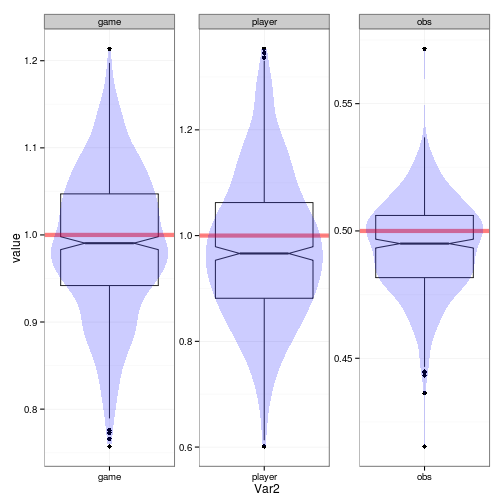# Power/assessment of Poisson GLMMs

Martí Casals writes:

The final data employed for the analysis were composed of 2187 cases (27 players * 81 games), having followed a filtering process. I dealt with a balanced study design with repeated measurements given that each player was observed the same number of games, and therefore the player was considered as a random effect. We carried out two mixed models, a Linear Mixed Model (LMM) through the nlme library (Pinheiro) and a Poisson mixed model with lme4 package (Bates).

``````library(lme4.0)  ## development version ...
``````
``````## Loading required package: Matrix
``````
``````## Loading required package: lattice
``````
``````library(plyr)
library(ggplot2)
theme_set(theme_bw())
library(reshape2)
set.seed(101)
``````
``````simdata <- function(nplayers = 27, ngames = 81, sd.game = 1, sd.player = 1,
sd.obs = 0.5, beta0 = 0.5) {
d <- expand.grid(player = factor(seq(nplayers)), game = factor(seq(ngames)))
u.game <- rnorm(ngames, sd = sd.game)
u.player <- rnorm(nplayers, sd = sd.player)
u.obs <- rnorm(ngames * nplayers, sd = sd.obs)
d <- transform(d, obs = factor(seq(nrow(d))), eta = beta0 + u.game[game] +
u.player[player] + u.obs)
d\$y <- rpois(nrow(d), exp(d\$eta))
d
}
``````
``````d1 <- simdata()
fit1 <- glmer(y ~ (1 | player) + (1 | game) + (1 | obs), data = d1, family = poisson)
``````
``````## Number of levels of a grouping factor for the random effects is *equal* to
## n, the number of observations
``````
``````unlist(VarCorr(fit1))
``````
``````##    obs   game player
## 0.2345 0.9652 1.0449
``````
``````simfun <- function(m = fit1, ...) {
sqrt(unlist(VarCorr(refit(m, simdata(...)\$y))))
}
s0 <- simfun()
``````
``````nsim <- 500
simres <- raply(nsim, simfun())
``````
``````summary(simres)
``````
``````##       obs             game           player
##  Min.   :0.415   Min.   :0.757   Min.   :0.601
##  1st Qu.:0.482   1st Qu.:0.942   1st Qu.:0.881
##  Median :0.495   Median :0.991   Median :0.966
##  Mean   :0.494   Mean   :0.991   Mean   :0.976
##  3rd Qu.:0.506   3rd Qu.:1.047   3rd Qu.:1.062
##  Max.   :0.572   Max.   :1.214   Max.   :1.353
``````
``````library(proto)
source("geom-hboxplot.r")
m <- transform(melt(simres), Var2 = factor(Var2, levels = c("game", "player",
"obs")))
truevals <- data.frame(Var2 = c("game", "obs", "player"), value = c(1, 0.5,
1))
ggplot(m, aes(x = Var2, y = value)) + geom_boxplot(notch = TRUE) + facet_wrap(~Var2,
scale = "free") + geom_hline(aes(yintercept = value), data = truevals, lwd = 2,
alpha = 0.5, colour = "red") + geom_violin(alpha = 0.2, colour = NA, fill = "blue")
``````This looks reasonably good on the whole, although the estimates are slightly biased (i.e. notches don't include true values for `player` and `obs`); this could be a finite-size/REML-y issue?

Would be nice to turn the whole thing sideways, but: `ggplot2 does not currently support free scales with a non-cartesian coord or coord_flip.`